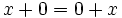Variety of Lie rings

Definition

As a plain variety

The variety of Lie rings is the variety of algebras with the operator domain consisting of:

• A binary operation$+$
• A unary operation$-$ (prefix symbol)
• A constant$0$
• A binary operation$[ \ , \ ]$

such that the following universal identities are satisfied:

1.$(x + y) + z = x + (y + z)$
2.$x + 0 = 0 + x$
3.$x + (-x) = (-x) + x = 0$
4.$x + y = y + x$
5.$[x,y+z] = [x,y] + [x,z]$
6.$[x + y,z] = [x,z] + [y,z]$
7.$[x,x] = 0$
8.$[x,[y,z]] + [y,[z,x]] + [z,[x,y]] = 0$

The identities (1)-(4) say that we get an Abelian group under$+$, the identities (5) and (6) say the Lie bracket is bilinear, the identity (7) says it is alternating, and the identity (8) is the Jacobi identity.

Properties

A complete listing of the universal algebra-theoretic properties of Lie rings is available at: